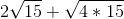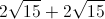# PSAT Math : How to factor a common factor out of squares

## Example Questions

### Example Question #4 : Squaring / Square Roots / Radicals

x2 = 36

Quantity A: x

Quantity B: 6

The relationship cannot be determined from the information given

Quantity B is greater

Quantity A is greater

The two quantities are equal

The relationship cannot be determined from the information given

Explanation:

x2 = 36 -> it is important to remember that this leads to two answers.

x = 6 or x = -6.

If x = 6: A = B.

If x = -6: A < B.

Thus the relationship cannot be determined from the information given.

### Example Question #5 : Squaring / Square Roots / Radicals

According to Heron's Formula, the area of a triangle with side lengths of a, b, and c is given by the following: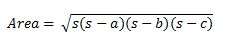where s is one-half of the triangle's perimeter.

What is the area of a triangle with side lengths of 6, 10, and 12 units?

4√14

14√2

8√14

12√5

48√77

8√14

Explanation:

We can use Heron's formula to find the area of the triangle. We can let a = 6, b = 10, and c = 12.

In order to find s, we need to find one half of the perimeter. The perimeter is the sum of the lengths of the sides of the triangle.

Perimeter = a + b + c = 6 + 10 + 12 = 28

In order to find s, we must multiply the perimeter by one-half, which would give us (1/2)(28), or 14.

Now that we have a, b, c, and s, we can calculate the area using Heron's formula.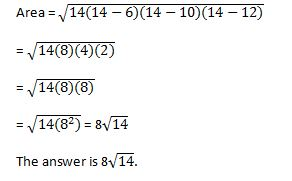### Example Question #2 : Squaring / Square Roots / Radicals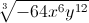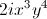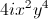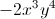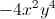Explanation:Look for perfect cubes within each term. This will allow us to factor out of the radical.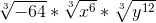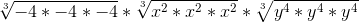Simplify.### Example Question #3 : Squaring / Square Roots / Radicals

Simplify the expression.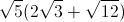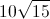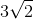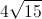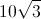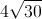Explanation:

Use the distributive property for radicals.Multiply all terms by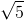.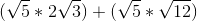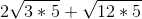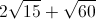Look for perfect square factors under each radical.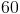has a perfect square of. Thecan be factored out.